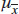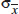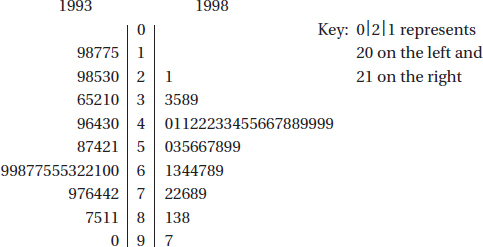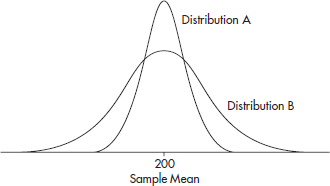# AP Statistics Multiple-Choice Practice Test 37

### Test Information10 questions23 minutes

1. The mean and standard deviation of the population {1, 5, 8, 11, 15} are μ = 8 and σ = 4.8, respectively. Let S be the set of the 125 ordered triples (repeats allowed) of elements of the original population. Which of the following is a correct statement about the meanand standard deviationof the means of the triples in S?

2. Consider the following studies being run by three different AP Statistics instructors.

I.One rewards students every day with lollipops for relaxation, encouragement, and motivation to learn the material.

II.One promises that all students will receive A's as long as they give their best efforts to learn the material.

III.One is available every day after school and on weekends so that students with questions can come in and learn the material.

3. A survey to measure job satisfaction of high school mathematics teachers was taken in 1993 and repeated 5 years later in 1998. Each year a random sample of 50 teachers rated their job satisfaction on a 1-to-100 scale with higher numbers indicating greater satisfaction. The results are given in the following back-to-back stemplot.What is the trend from 1993 to 1998 with regard to the standard deviation and range of the two samples?

4. The number of days it takes to build a new house has a variance of 386. A sample of 40 new homes shows an average building time of 83 days. With what confidence can we assert that the average building time for a new house is between 80 and 90 days?

5. A shipment of resistors have an average resistance of 200 ohms with a standard deviation of 5 ohms, and the resistances are normally distributed. Suppose a randomly chosen resistor has a resistance under 194 ohms. What is the probability that its resistance is greater than 188 ohms?

6. Suppose 4% of the population have a certain disease. A laboratory blood test gives a positive reading for 95% of people who have the disease and for 5% of people who do not have the disease. If a person tests positive, what is the probability the person has the disease?

7. For which of the following is it appropriate to use a census?

8. On the same test, Mary and Pam scored at the 64th and 56th percentiles, respectively. Which of the following is a true statement?

9. Which of the following is a true statement?

10. The random variable describing the number of minutes high school students spend in front of a computer daily has a mean of 200 minutes. Samples of two different sizes result in sampling distributions with the two graphs below.Which of the following is a true statement?